##全国校区# 原理+代码|Python实战多元线性回归模型2020-08-05

# 前言

「多元线性回归模型」非常常见，是大多数人入门机器学习的第一个案例，尽管如此，里面还是有许多值得学习和注意的地方。其中多元共线性这个问题将贯穿所有的机器学习模型，所以本文会「将原理知识穿插于代码段中」，争取以不一样的视角来叙述和讲解「如何更好的构建和优化多元线性回归模型」。主要将分为两个部分：

• 详细原理
• Python 实战

# Python 实战

Python 多元线性回归的模型的实战案例有非常多，这里虽然选用的经典的房价预测，但贵在的流程简洁完整，其中用到的精度优化方法效果拔群，能提供比较好的参考价值。

# 数据探索

```import pandas as pd
import numpy as np
import seaborn as sns
import matplotlib.pyplot as plt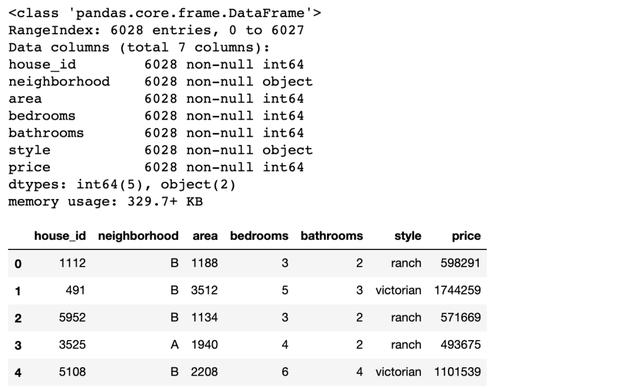• neighborhood/area:所属街区和面积
• bedrooms/bathrooms:卧室和浴室
• style:房屋样式

# 多元线性回归建模

```from statsmodels.formula.api import ols
# 小写的 ols 函数才会自带截距项，OLS 则不会
# 固定格式：因变量 ~ 自变量(+ 号连接)
lm = ols('price ~ area + bedrooms + bathrooms', data=df).fit()
lm.summary()```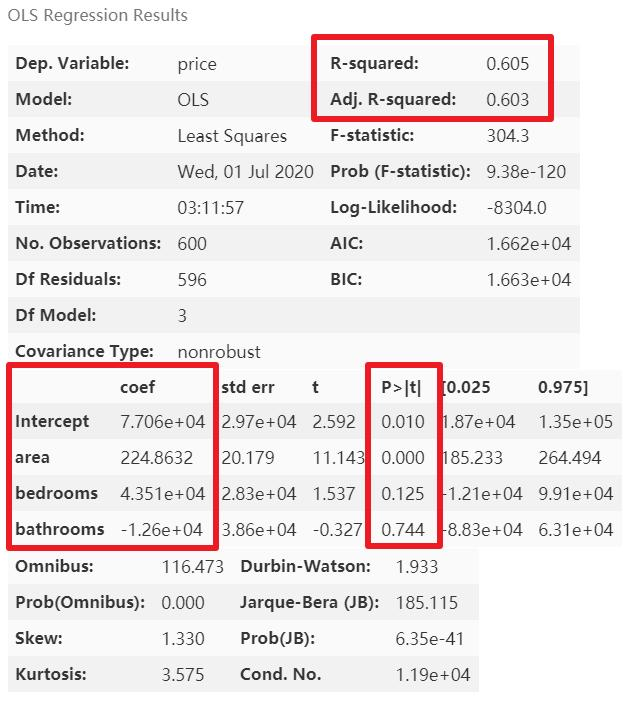# 模型优化

```# 类别变量，又称为名义变量，nominal variables
nominal_vars = ['neighborhood', 'style']

for each in nominal_vars:
print(each, ':')
print(df[each].agg(['value_counts']).T)  # Pandas 骚操作
# 直接 .value_counts().T 无法实现下面的效果
## 必须得 agg，而且里面的中括号 [] 也不能少
print('='*35)```

# 虚拟变量的设置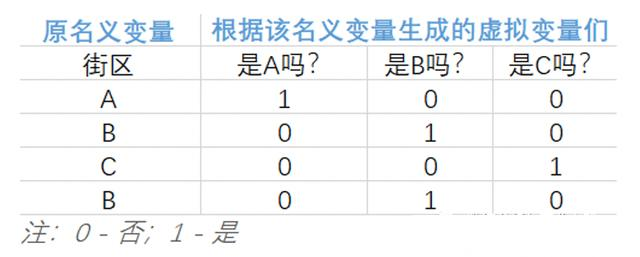• 该名义变量有 n 类，就能拆分出 n 个虚拟变量
• 巧妙的使用 0 和 1 来达到「用虚拟变量列代替原名义变量所在类别」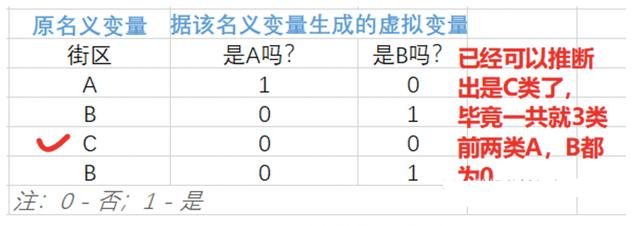• 抽出希望转换的名义变量(一个或多个)
• pandas的get_dummies函数
• 与原数据集横向拼接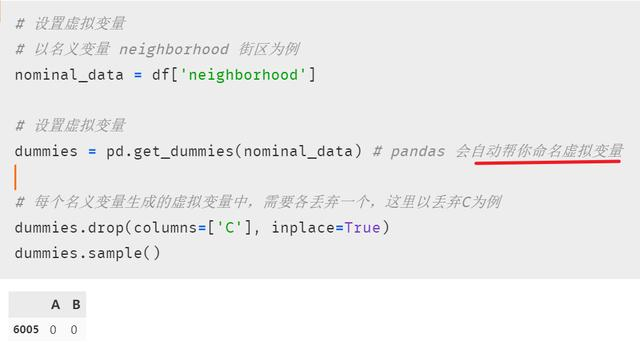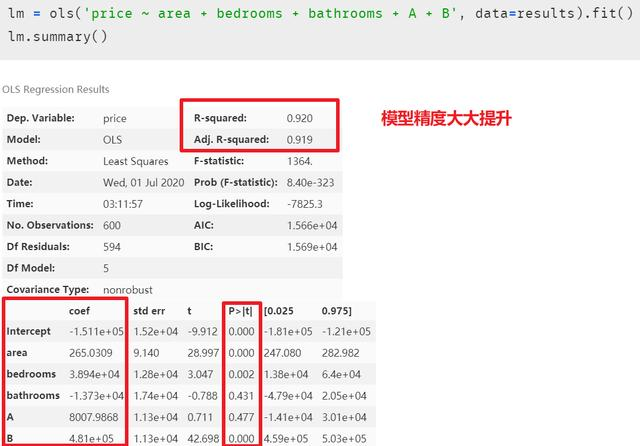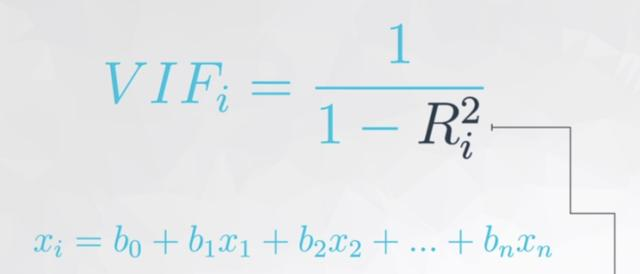• 每个自变量都会有一个膨胀因子值 ，最后根据值的大小来选择是否删减
• 「既然  表示相关性，是谁跟谁的相关性呢?」 是自变量中的某一变量与除它外剩余的自变量进行多元线性回归，取回归结果，即模型精度来作为这个变量与剩余自变量的相关性。听起来可能有点绕，这里举一下实例(用 “面积、卧室数量和浴室数量” 作为自变量来预测房价，在进行自变量的方差膨胀因子的检测时，面积、卧室数和浴室数轮流做单独的因变量，剩下的两个变量作为自变量，来看看这三个自变量中那个变量对其余两个变量的解释性高)
•  越大，如已经到了 0.9，那分母就很小， 的值就等于 10，即表示这个自变量已经同时解释了另外的某个或多个自变量，存在多元共线性，可以考虑删除一些自变量。

越大，显示共线性越严重。经验判断方法表明：「当 ，不存在多重共线性；当 ，存在较强的多重共线性；当 ，存在严重多重共线性」

# 方差膨胀因子的检测

```def vif(df, col_i):
"""
df: 整份数据
col_i：被检测的列名
"""
cols = list(df.columns)
cols.remove(col_i)
cols_noti = cols
formula = col_i + '~' + '+'.join(cols_noti)
r2 = ols(formula, df).fit().rsquared
return 1. / (1. - r2)```

```test_data = results[['area', 'bedrooms', 'bathrooms', 'A', 'B']]
for i in test_data.columns:
print(i, '\t', vif(df=test_data, col_i=i))```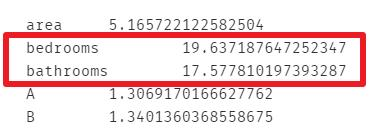```lm = ols(formula='price ~ area + bathrooms + A + B', data=results).fit()
lm.summary()```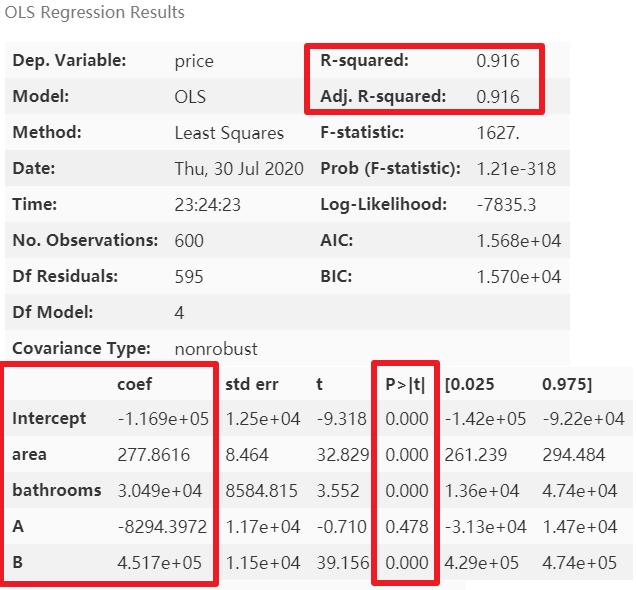```test_data = results[['area', 'bedrooms', 'A', 'B']]
for i in test_data.columns:
print(i, '\t', vif(df=test_data, col_i=i))```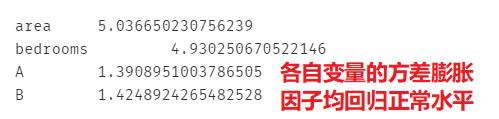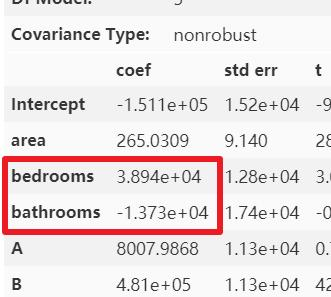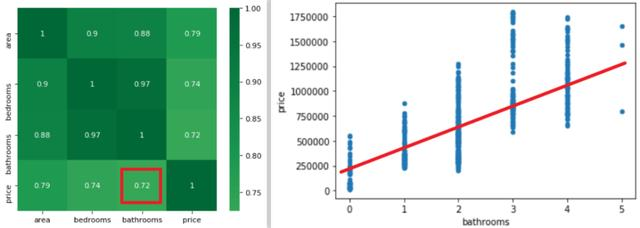# 模型解释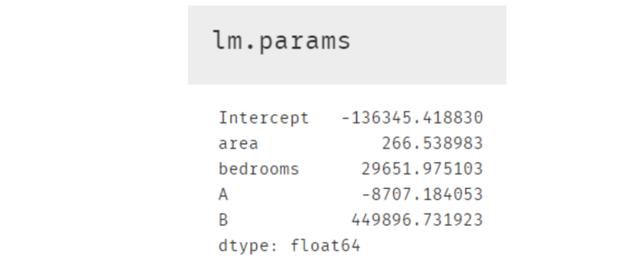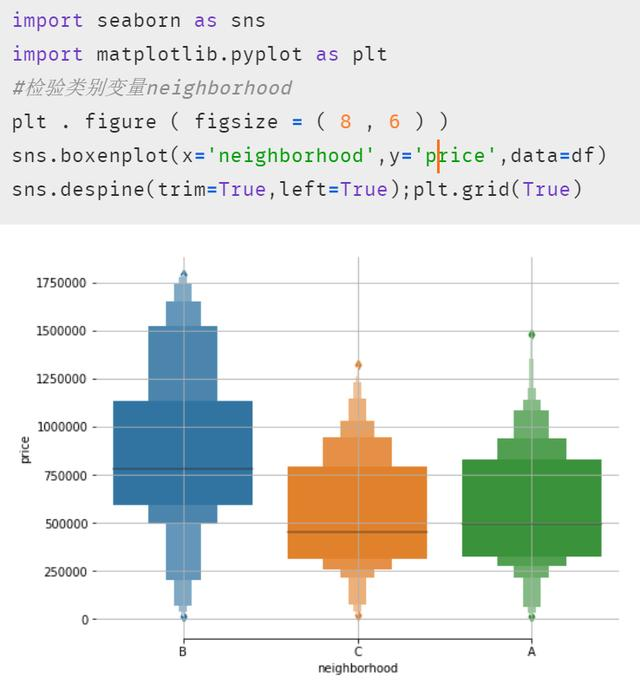# 小结

OK